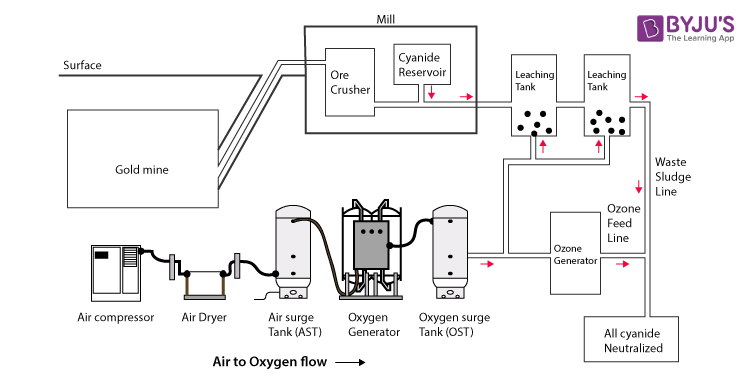Win up to 100% scholarship on Aakash BYJU'S JEE/NEET courses with ABNAT Win up to 100% scholarship on Aakash BYJU'S JEE/NEET courses with ABNAT

# Cyanide Process

The cyanide process, which is also known as the Macarthur-forest process, is widely used in extracting gold or silver from the ores by dissolving them in a dilute solution of potassium cyanide or sodium cyanide. This process was introduced in the year 1887 by Scottish chemists named Robert W. Forrest, John S. MacArthur and William Forrest.

This method involves three main steps. In the first step, the finely ground ore is contacted with the solution containing the cyanide, the second step involves the separation of solids from the clear solution, and the third step involves the recovery of precious metals from the solution by precipitating with the zinc dust.

## Cyanide Process – Extraction of Gold through CyanidationGold is usually found in low concentration in the form of ore, which is mined. Gold should be separated from other minerals present in the ore. As gold is insoluble, it should be separated from other minerals to make it soluble. Hence, sodium cyanide can be added, where cyanide ions form a complex ion with the molecules of gold.

The cyanide process is explained below.

• The ore is grounded and crushed. If the ore containing the gold has other forms of metals or any kind of sulphide minerals, then it requires additional treatments before going through the process of leaching. The gold is mixed with the sodium cyanide, which leads to the following Elsener’s equation and reaction. This is done to make soluble gold.
• Now, the gold is soluble. This method of making soluble gold is known as leaching. In the process of leaching, a dilute form of sodium cyanide is added to the ore containing the gold. Since gold is soluble after the leaching process, it is free to move through the membrane, while the rest of the ore cannot pass through the membrane. Lime is added to sodium cyanide to make the pH between 10-11 to favour the reactants and bring them to equilibrium.
• The slurry formed is treated with activated zinc or carbon for the extraction of gold. Cementation is the next process which involves the use of a zinc electrode with a paste of carbon immersed in the solution containing the gold cyanide.

### Reactions Involved

The following reactions occur:

At cathode –

$$\begin{array}{l}e^{-} + Au(CN)_{2}]^{-}\rightarrow Au + 2CN^{-}\end{array}$$

Gold is thus reduced by gaining electrons or by the decrease in the oxidation number.

At Anode –

$$\begin{array}{l}Zn + 2OH^{-}\rightarrow Zn(OH)_{2} + 2e^{-}\end{array}$$

Zinc is next oxidised to lose electrons, or there is an increase in the oxidation number.

$$\begin{array}{l}Zn(OH)_{2} + 4CN^{-}\rightarrow Zn(CN)_{4}^{2-} + 2OH^{-}\end{array}$$

The cyanide remains in the tailings, i.e., the slurry obtained after the process of gold leaching needs to be recycled or destroyed in some way.

Test Your Knowledge on Cyanide Process